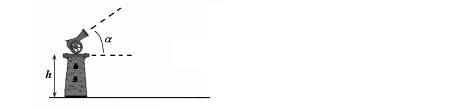Problem: A cannon is placed on a tower an UNKNOWN height h from the ground. The cannon is inclined an angle α from the horizontal when a ball is fired. Assuming that there is no friction, that the initial speed of the ball is v0, and that the ball lands a distance L from the base of the tower: a) How high above ground does the ball go? b) What is the speed of the ball at the maximum height? c) What is the height h of the tower? If needed, you can assume you know h for parts (a) and (b). Write your results in terms of α, v  0, L, g, and h (only for parts a and b). Remember to check the dimensions/units for each answer.

87% (18 ratings)
Problem Details

A cannon is placed on a tower an UNKNOWN height h from the ground. The cannon is inclined an angle α from the horizontal when a ball is fired. Assuming that there is no friction, that the initial speed of the ball is v0, and that the ball lands a distance L from the base of the tower:

a) How high above ground does the ball go?

b) What is the speed of the ball at the maximum height?

c) What is the height h of the tower?

If needed, you can assume you know h for parts (a) and (b). Write your results in terms of α, v  0, L, g, and h (only for parts a and b). Remember to check the dimensions/units for each answer.